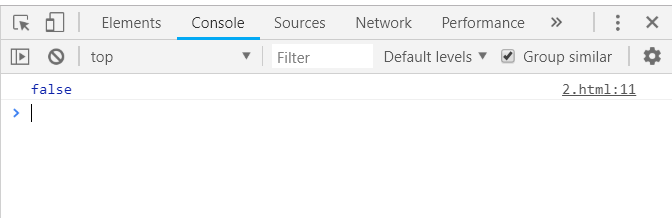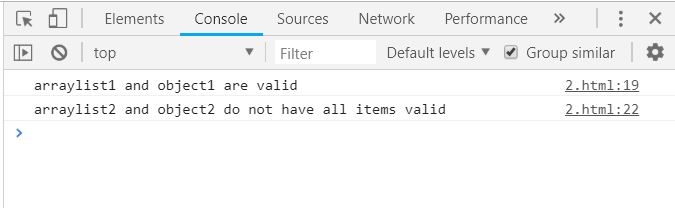Open in App
Not now

# Underscore.js _.every Function

• Last Updated : 26 Oct, 2022

The Underscore.js is a JavaScript library that provides a lot of useful functions that helps in the programming in a big way like the map, filter, invoke etc even without using any built-in objects. The _.every() function is used to test all the elements of the list can pass the given test. It stops and returns ‘false’ if at least one element is not fulfill the given test. When all the elements of the list are passed to the function/iterate and no more elements remains then the _.every function to traverse and the value false has not yet returned as answer then return true as final answer. Pass the numbers, characters, array, objects etc to the _.every function. Also, one can use to _.every() function together like inside an if loop etc.

Syntax:

`_.every(list, [predicate], [context])`

Parameters: This function accepts three parameters as mentioned above and described below:

• List: This parameter is used to set the list of elements.
• Predicate: This parameter is used to test the condition.
• Context: This parameter is used to display the content.

Return values: The returned value which is either ‘true’ ( when every element of the list fulfills the given condition) or ‘false’ ( when at least one element does not fulfill the condition) Passing an array to the _every function(): The ._every() function takes the element from the list one by one and do the specified operations on the code. Below example contains the operation to find all the elements of the list that are valid or not. Valid means that they do not contain Null, Blanks, false etc. After traversing and checking all the elements, the every function ends. Here even if a single element is not valid then also the answer is false.

Example:

## html

 `<``html``>` `    ``<``head``>` `        ``<``script` `type="text/javascript" src = ` `        ``"https://cdnjs.cloudflare.com/ajax/libs/underscore.js/1.9.1/underscore-min.js">` `        ``` `        ``<``script` `type="text/javascript" src = ` `        ``"https://cdnjs.cloudflare.com/ajax/libs/underscore.js/1.9.1/underscore.js">` `    ``  ` `    ``<``body``>` `        ``<``script` `type="text/javascript">` `              ``var arrayvalues = [false, true, 'yes', null, 1];` `              ``console.log(_.every(arrayvalues, function (value) {` `                  ``return (value);` `              ``}));` `        ``` `    `` ` ``

Output:Passing a list of numbers to _.every() function: Pass a list of numbers and do the simple operations on it. Below example is used to find whether a number is even or not. If all the numbers in the list are even then the output is true otherwise false. Example:

## html

 `<``html``>  ` `    ``<``head``>` `        ``<``script` `type="text/javascript" src = ` `        ``"https://cdnjs.cloudflare.com/ajax/libs/underscore.js/1.9.1/underscore-min.js">` `        ``` `        ``<``script` `type="text/javascript" src = ` `        ``"https://cdnjs.cloudflare.com/ajax/libs/underscore.js/1.9.1/underscore.js">` `        ``` `    ``      ` `    ``<``body``>` `        ``<``script` `type="text/javascript">` `            ``console.log(_.every([2, 4, 5], function(num) { return num % 2 == 0; }));` `        ``` `    `` ` ``

Output:Passing a structure to the _.every() function: First declare the array (The name of array is people). Choose one condition to check hasLongHairs. Console.log display the final answer. Example:

## html

 `<``html``>  ` `    ``<``head``>` `        ``<``script` `type="text/javascript" src = ` `        ``"https://cdnjs.cloudflare.com/ajax/libs/underscore.js/1.9.1/underscore-min.js">` `        ``` `        ``<``script` `type="text/javascript" src = ` `        ``"https://cdnjs.cloudflare.com/ajax/libs/underscore.js/1.9.1/underscore.js">` `        ``` `    ``      ` `    ``<``body``>` `        ``<``script` `type="text/javascript">` `            ``var people = [` `                ``{name: 'sakshi', car: ''},` `                ``{name: 'aishwarya', car: true},` `                ``{name: 'akansha', car: true},` `                ``{name: 'preeti', car: true}` `            ``],` `      `  `            ``hasLongHairs = function (value) {` `                ``return (value.car !== '');` `            ``};` `     `  `            ``console.log(_.every(people, hasLongHairs));` `        ``` `    `` ` ``

Output:Using two _.every() function together: Pass different objects to each _.every() function and then use the following results together by using the logical operators like &&, ||, ! etc. Here, the object1 and arralist1 contains all the true values so the resultant of two true will also be true. Hence, first condition is satisfied. The object2 contains ‘null’ and arraylist2 also contains ‘null’ so they are not valid. Use ‘!’ before every _.every() function so the resultant are two true values. Example:

## html

 `<``html``>  ` `    ``<``head``>` `        ``<``script` `type="text/javascript" src = ` `        ``"https://cdnjs.cloudflare.com/ajax/libs/underscore.js/1.9.1/underscore-min.js">` `        ``` `        ``<``script` `type="text/javascript" src = ` `        ``"https://cdnjs.cloudflare.com/ajax/libs/underscore.js/1.9.1/underscore.js">` `        ``` `    ``      ` `    ``<``body``>` `        ``<``script` `type="text/javascript">` `             ``var arraylist1 = [true];` `             ``var arraylist2 = [null, {}, undefined, {}];` `             ``var object1 = {prop1: true};` `             ``var object2 = {` `                    ``prop1: null,` `                    ``prop2: true, prop3: true,` `             ``}; ` `             ``if (_.every(arraylist1) && _.every(object1)) {` `                    ``console.log('arraylist1 and object1 are valid');` `             ``}` `             ``if (!_.every(arraylist2) && !_.every(object2)) {` `                    ``console.log('arraylist2 and object2 do not have all items valid');` `             ``}` `        ``` `    `` ` ``

Output:My Personal Notes arrow_drop_up
Related Articles